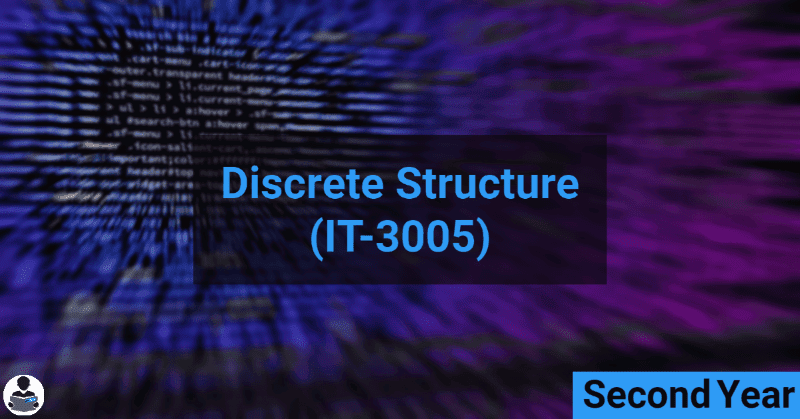Discrete Structures (IT-3005) - B.E RGPV CBCS & CBGS Scheme Notes -->
##### Discrete Structures (IT-3005)## Course objectives

The main objectives of this course are:
1. To introduce students with sets, relations, functions, graph, and probability.
2. Students can perform set operation and solve logical reasoning and verify the correctness of logical statement.
3. They can apply the properties of relations and find partially ordered set and lattices.

## Syllabus

UNIT 1:
Set Theory, Relation, Function, Theorem Proving Techniques : Set Theory: Definition of sets, countable and uncountable sets, Venn Diagrams, proofs of some general identities on sets Relation: Definition, types of relation, composition of relations, Pictorial representation of relation, Equivalence relation, Partial ordering relation, Job Scheduling problem Function: Definition, type of functions, one to one, into and onto function, inverse function, composition of functions, recursively defined functions, pigeonhole principle. Theorem proving Techniques: Mathematical induction, Proof by contradiction.

UNIT 2:
Set Theory, Relation, Function, Theorem Proving Techniques : Set Theory: Definition of sets, countable and uncountable sets, Venn Diagrams, proofs of some general identities on sets Relation: Definition, types of relation, composition of relations, Pictorial representation of relation, Equivalence relation, Partial ordering relation, Job Scheduling problem Function: Definition, type of functions, one to one, into and onto function, inverse function, composition of functions, recursively defined functions, pigeonhole principle. Theorem proving Techniques: Mathematical induction, Proof by contradiction.

UNIT 3:
Set Theory, Relation, Function, Theorem Proving Techniques : Set Theory: Definition of sets, countable and uncountable sets, Venn Diagrams, proofs of some general identities on sets Relation: Definition, types of relation, composition of relations, Pictorial representation of relation, Equivalence relation, Partial ordering relation, Job Scheduling problem Function: Definition, type of functions, one to one, into and onto function, inverse function, composition of functions, recursively defined functions, pigeonhole principle. Theorem proving Techniques: Mathematical induction, Proof by contradiction.

UNIT 4:
Set Theory, Relation, Function, Theorem Proving Techniques : Set Theory: Definition of sets, countable and uncountable sets, Venn Diagrams, proofs of some general identities on sets Relation: Definition, types of relation, composition of relations, Pictorial representation of relation, Equivalence relation, Partial ordering relation, Job Scheduling problem Function: Definition, type of functions, one to one, into and onto function, inverse function, composition of functions, recursively defined functions, pigeonhole principle. Theorem proving Techniques: Mathematical induction, Proof by contradiction.

UNIT 5:
Posets, Hasse Diagram and Lattices: Introduction, ordered set, Hasse diagram of partially, ordered set, isomorphic ordered set, well ordered set, properties of Lattices, bounded and complemented lattices. Combinatorics: Introduction, Permutation and combination, Binomial Theorem, Recurrence Relation and Generating Function: Introduction to Recurrence Relation and Recursive algorithms , Linear recurrence relations with constant coefficients, Homogeneous solutions, Particular solutions, Total solutions , Generating functions , Solution by method of generating functions.

• Unit 1
• Unit 2
• Unit 3
• Unit 4
• Unit 5

## Course Outcomes

On completion of the course;
1. Students will be able to understand the notion of mathematical thinking, and algorithmic thinking and be able to apply them in problem solving such as formal specification, verification, and basic concepts of set theory.
2. Students understand the basic principle of Boolean algebra, logic and set theory.
3. Be able to construct simple mathematical proof and possess the ability to verify them.

## Reference Books:

1. C.L.Liu” Elements of Discrere Mathematics” TMH.
2. Lipschutz, “Discrete mathematics (Schaum)”,TMH.
3. U.S Gupta “ Discrete Mathematical Structures” Pearson.
4. S. Santha,” Discrete Mathematics with Combinatorics and graph theory”, Cengage Learning.
5. Dr.Sukhendu. Dey “ Graph Theory With Applications” Shroff Publishers

## You May Also Like

#### Services

###### COMPLETELY FREE !!!

Yup, everything is free....

###### NO REGISTRATION REQUIRED

User doesn't have to register for accessing the files, all the files are free & universally accessible without any condition or restriction.

###### RESPONSIVE DESIGN & USER-FRIENDLY

Our webpages are responsive & user-friendly, which means it will automatically adjust according to your device screen size and you will find stuff without ant hustle.

All the files are uploaded on our super-fast servers so that they can be easily downloaded with high speed.

###### NEW PROJECTS

For providing a better experience to our users we are developing our Android application, the application will have a lot of awesome features so stay tuned ;).

###### AWESOME SUPPORT TEAM

Our AI-powered Chatbots are always here to help you so, feel free to ask any question or report if you face any problem. Our team also monitors all chatbots traffic & they will contact you if chatbot fails to help.